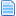# Show content

This section allows you to browse the content for this member. Note that you can only see content for which you have sufficient viewing permissions.

User### Re: Blob rivals disappear during battle

November 12, 2019, 12:00:21 pm View in topic contextPosted by Shadow_Harlequin  in Blob rivals disappear during battle (Started by Shadow_Harlequin November 12, 2019, 08:03:30 am
Board: M.U.G.E.N Development Help

This happens with every enemy of Blob. Not always, sometimes the battle ends successfully, sometimes there is a mistake. Here is the debug mode ... I have little sense in all of this.

https://ibb.co/dkbWwpT image

;--------------------------------------segundo player sendo engolido
[Statedef 902]
type    = A
movetype= H
physics = N
ctrl = 0

;animaзгo do p2 sendo engolido
[State 902, 1]
type = ChangeAnim2
Trigger1 = Time = 0
value = 804

;nгo movimentar tela
[State  902,ntela]
type = ScreenBound
trigger1 = 1
value = 0
movecamera = 1,0

[State 902, n2tela]
type = screenbound
Trigger1 = 1
value = 0### Blob rivals disappear during battle

November 12, 2019, 08:03:30 am View in topic contextPosted by Shadow_Harlequin  in Blob rivals disappear during battle (Started by Shadow_Harlequin November 12, 2019, 08:03:30 am
Board: M.U.G.E.N Development Help

Hello, I'm a newbie. The character's rival Blob periodically disappears during an autoboy. Perhaps during an attempt to "swallow". Here's a video

Code:
`;-----------------------------------------------Arresso 2 (comer);---------------------------------------inicio[Statedef 1901]type    = Smovetype= Aphysics = Sjuggle  = 5velset = 0,0poweradd = 20anim = 1901ctrl = 0[State 1901, pega] ;Perto do oponentetype = HitDeftrigger1 = AnimElem = 1attr = S, NTanimtype  = lowdamage    = 20pausetime = 0,3sparkxy = 0, 260ground.type = lowp1stateno = 801p2stateno = 902ground.slidetime = 2 ground.hittime  = 20ground.velocity = -1 [State 1901, 2]type = ChangeStatetrigger1 = AnimTime = 0value = 0ctrl = 1;-----------------------------------Pegando[Statedef 801] type    = Smovetype= Aphysics = Sanim = 8011sprpriority = -1poweradd = 75[State 801, Bind]type = TargetBindtrigger1 = animelem = 1, >=0trigger1 = animelem = 2, < 0pos = 10, -0time = 1[State 801, Bind]type = TargetBindtrigger1 = animelem = 3, >=0trigger1 = animelem = 4, < 0pos = 10, -0;-60time = 1[State 801, Bind]type = TargetBindtrigger1 = animelem = 5, >=0trigger1 = animelem = 6, < 0pos = 13,-80time = 1[State 801, Bind]type = TargetBindtrigger1 = animelem = 7pos = 2, 0time = 1[State 801, liftoff]type = sprpriorityTrigger1 = animelem = 7value = 2;aciona o state invisivel para p2[State 801, invisp2]type = TargetStateTrigger1 = animelem = 7value = 9011ctrl = 0[State 801, mast]type = ChangeStatetrigger1 = animelem = 8value = 803;-----------------------------------------------------mastigando[Statedef 803]type    = Amovetype= A ;Get hitphysics = Nanim = 803ctrl = 0[state 1, varclay]type = varsettriggerall = var(2) >=1trigger1 = time = 0v = 30value = 1;dentro da barriga[State 803, som]type = playsndtrigger1 = time = 0value = 0,8[State 803, 1]type = screenboundTrigger1 = 1value = 0;se o adversбrio for atingido volta ao normal[State 803, normal]type = ChangeStateTrigger1 = P2MoveType = Hvalue = 0ctrl=1[State 803, cuspe]type = ChangeStateTrigger1 = animelem = 13value = 805;--------------------------------------segundo player sendo engolido[Statedef 902]type    = Amovetype= H physics = Nctrl = 0;animaзгo do p2 sendo engolido[State 902, 1]type = ChangeAnim2Trigger1 = Time = 0value = 804;nгo movimentar tela[State  902,ntela]type = ScreenBoundtrigger1 = 1value = 0movecamera = 1,0 [State 902, n2tela]type = screenboundTrigger1 = 1value = 0;----------------------------------------p2 imovel,invisivel e cria uma caixa de colisгo[Statedef 9011]type    = Smovetype= I;Hphysics = S;Njuggle  = 1velset = 0,0ctrl = 0[State  9011,ntela]type = ScreenBoundtrigger1 = 1value = 0movecamera = 1,0 [State 9011, 1]type = Changeanim2trigger1 = time = 0value = 9011;------------------------------------------final do arremesso usando um hit, para fazer p2 voltar ao normal[Statedef 805]type    = Amovetype= Aphysics = Cjuggle  = 7poweradd= 70ctrl = 0anim = 450sprpriority = 2[State 805, 2]type = PlaySndtrigger1 = Time = 2value = 3,8[State 805, 3]type = HitDeftriggerall =var(57)=0trigger1 = Time = 0attr = S, NTdamage    = 102hitflag = MAFDguardflag = Lpausetime = 0,3sparkno = -1sparkxy = 0,0;;hitsound   = 5,2;guardsound = 6,0ground.type = Tripground.slidetime = 10ground.hittime  = 15ground.velocity = -1.5,-10air.velocity = -1.2,-3guard.velocity = -5fall = 1[State 805, 3]type = HitDeftriggerall =var(57)=1trigger1 = Time = 0attr = S, NTdamage    = 85hitflag = MAFDguardflag = Lpausetime = 0,3sparkno = -1sparkxy = 0,0;;hitsound   = 5,2;guardsound = 6,0ground.type = Tripground.slidetime = 10ground.hittime  = 15ground.velocity = -1.5,-10air.velocity = -1.2,-3guard.velocity = -5fall = 1kill = 0[State 805, 4]type = ChangeStatetrigger1 = AnimTime = 0value = 0ctrl = 1`

I also use such code in the cmd file ...

[State -1, AI Hyper Tatsumaki]
type = changestate
triggerall = (roundstate = 2) && Power >=1
triggerall = (statetype != A) && (p2statetype != L)
trigger1 = (Ctrl) && (random = [0,160])
trigger2 = ((Stateno = 640) || (Stateno = 650)) && MoveHit
trigger3 = (Stateno = 1300) && (AnimElem = 11) && MoveHit
value = 1901

With statetype = S it periodically rises into the air. Stateno also tried to change